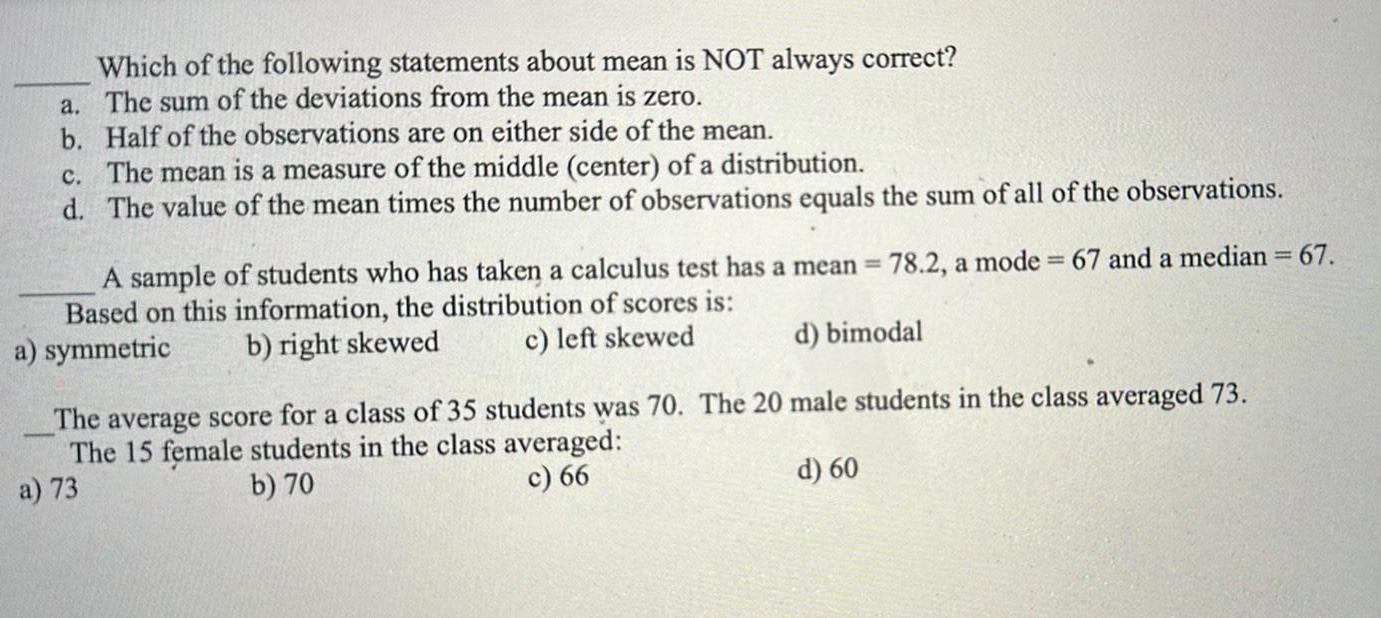Question:

# Which of the following statements about mean is NOT always

Last updated: 9/18/2023Which of the following statements about mean is NOT always correct a The sum of the deviations from the mean is zero b Half of the observations are on either side of the mean c The mean is a measure of the middle center of a distribution d The value of the mean times the number of observations equals the sum of all of the observations 78 2 a mode 67 and a median 67 A sample of students who has taken a calculus test has a mean Based on this information the distribution of scores is c left skewed b right skewed a symmetric d bimodal The average score for a class of 35 students was 70 The 20 male students in the class averaged 73 The 15 female students in the class averaged a 73 b 70 c 66 d 60